# HCF and LCM

## Practise finding the highest common factor (HCF), sometimes called the greatest common divisor, and the lowest common multiple (LCM) of two numbers.

##### MenuTreesLevel 1Level 2Level 3Level 4Level 5Level 6Level 7HelpMore

This is level 2: Finding the lowest common multiple (LCM) of two numbers. You can earn a trophy if you get at least 9 correct and you do this activity online.

 Find the LCM of6 and 9.Find the LCM of2 and 4.Find the LCM of3 and 4.Find the LCM of4 and 12.Find the LCM of30 and 18.Find the LCM of36 and 27.Find the LCM of22 and 55.Find the LCM of56 and 40.Find the LCM of60 and 45.Find the LCM of72 and 27.Find the LCM of11 and 22.Find the LCM of68 and 85.Check

This is HCF and LCM level 2. You can also try:
Level 1 Level 3 Level 4 Level 5 Level 6 Level 7

## Instructions

Try your best to answer the questions above. Type your answers into the boxes provided leaving no spaces. As you work through the exercise regularly click the "check" button. If you have any wrong answers, do your best to do corrections but if there is anything you don't understand, please ask your teacher for help.

When you have got all of the questions correct you may want to print out this page and paste it into your exercise book. If you keep your work in an ePortfolio you could take a screen shot of your answers and paste that into your Maths file.

## Transum.org

This web site contains over a thousand free mathematical activities for teachers and pupils. Click here to go to the main page which links to all of the resources available.## More Activities:

Mathematicians are not the people who find Maths easy; they are the people who enjoy how mystifying, puzzling and hard it is. Are you a mathematician?

Comment recorded on the s /Indice 'Starter of the Day' page by Busolla, Australia:

"Thank you very much for providing these resources for free for teachers and students. It has been engaging for the students - all trying to reach their highest level and competing with their peers while also learning. Thank you very much!"

Comment recorded on the 7 December 'Starter of the Day' page by Cathryn Aldridge, Pells Primary:

"I use Starter of the Day as a registration and warm-up activity for my Year 6 class. The range of questioning provided is excellent as are some of the images.
I rate this site as a 5!"

Each month a newsletter is published containing details of the new additions to the Transum website and a new puzzle of the month.

The newsletter is then duplicated as a podcast which is available on the major delivery networks. You can listen to the podcast while you are commuting, exercising or relaxing.

Transum breaking news is available on Twitter @Transum and if that's not enough there is also a Transum Facebook page.

#### Strategy Games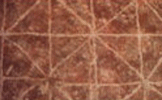We have collected together lots of strategy games for you to enjoy. Many of them have mathematical principles involved in their design so would make a good activity for a Mathematics lesson.

There are answers to this exercise but they are available in this space to teachers, tutors and parents who have logged in to their Transum subscription on this computer.

A Transum subscription unlocks the answers to the online exercises, quizzes and puzzles. It also provides the teacher with access to quality external links on each of the Transum Topic pages and the facility to add to the collection themselves.

Subscribers can manage class lists, lesson plans and assessment data in the Class Admin application and have access to reports of the Transum Trophies earned by class members.

Subscribe

## Go Maths

Learning and understanding Mathematics, at every level, requires learner engagement. Mathematics is not a spectator sport. Sometimes traditional teaching fails to actively involve students. One way to address the problem is through the use of interactive activities and this web site provides many of those. The Go Maths page is an alphabetical list of free activities designed for students in Secondary/High school.

## Maths Map

Are you looking for something specific? An exercise to supplement the topic you are studying at school at the moment perhaps. Navigate using our Maths Map to find exercises, puzzles and Maths lesson starters grouped by topic.

## Teachers

If you found this activity useful don't forget to record it in your scheme of work or learning management system. The short URL, ready to be copied and pasted, is as follows:

Alternatively, if you use Google Classroom, all you have to do is click on the green icon below in order to add this activity to one of your classes.

It may be worth remembering that if Transum.org should go offline for whatever reason, there are mirror sites at Transum.com and Transum.info that contain most of the resources that are available here on Transum.org.

When planning to use technology in your lesson always have a plan B!

Do you have any comments? It is always useful to receive feedback and helps make this free resource even more useful for those learning Mathematics anywhere in the world. Click here to enter your comments.For All:

Scan the QR code below to visit the online version of this activity.https://Transum.org/go/?Num=337

## Description of Levels

Sieve Use the Sieve of Eratosthenes to find prime numbers.

Factor Trees An interactive and very visual way to break down a number into its prime factors.

CloseLevel 1 - Finding the highest common factor (HCF) of two numbers.

Level 2 - Finding the lowest common multiple (LCM) of two numbers

Level 3 - Finding the highest common factor (HCF) of large numbers.

Level 4 - Finding the lowest common multiple (LCM) of large numbers

Level 5 - Finding the HCF and LCM of three numbers

Level 6 - Given the HCF and LCM find the numbers

Level 7 - Mixed application questions

HCF and LCM given An Advanced Lesson Starter.

Answers to this exercise are available lower down this page when you are logged in to your Transum account. If you don’t yet have a Transum subscription one can be very quickly set up if you are a teacher, tutor or parent.

## Help

The highest common factor (HCF) of two numbers is the largest number that divides exactly into both of the numbers.

You can Find the HCF of numbers by listing the prime factors of both numbers then multiplying together the factors that appear in both lists.

For example find the HCF of 24 and 36
24 = 2x2x2x3 and 36 = 2x2x3x3
so the HCF of 24 and 36 is 2x2x3 = 12

The lowest common multiple (LCM), or least common multiple, is the smallest number that both numbers divide into exactly.

You can Find the LCM of numbers by listing the prime factors of both numbers and then multiply all the prime factors of the larger number by those prime factors of the smaller number that are not already included.

For example find the LCM of 24 and 36
24 = 2x2x2x3 and 36 = 2x2x3x3
so the LCM of 24 and 36 is 2x2x3x3 x 2 = 72

## Venn Diagram

A Venn diagram may help you with the task of finding the HCF and LCM of 24 and 36.

Express each number as the primes which multiplied together would give you that number. Write them in Venn diagram sets: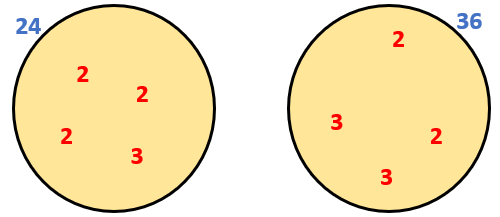Show the sets intersecting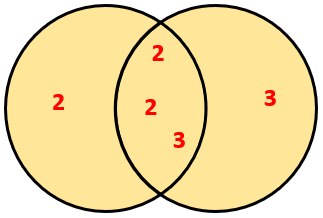Multiply the numbers in the intersection of the sets to find the HCF, 2x2x3 = 12.

Multiply all the numbers in the overlapping sets diagram to find the LCM, 2x2x2x3x3 = 72.

## The Indian Method

Click here to see an animated demonstration of this cool way to find both the HCF and LCM of two numbers.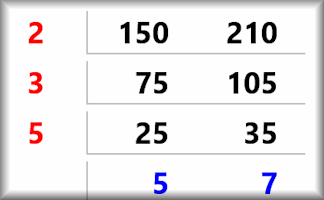## A Calculator Method

Advanced calculators have built in functions for finding the HCF and LCM of two numbers but there is a trick for finding the HCF using a modern scientific calculator.

If the two numbers are entered using the fraction template the calculator will express that fraction in its lowest terms. It does this by dividing numerator and denominator by their HCF.

For example to find the HCF of 24 and 36 enter 24/36 then press enter.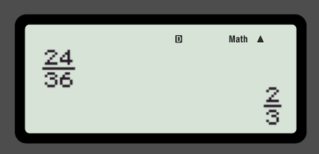Considering the denominators, we now need to find what 24 was divided by to give 2. So dividing 24 by 2 gives 12 which is the HCF.

## Connecting HCF and LCM

When you have found the HCF of the numbers a and b the LCM can be found using the following formula:

## Greatest Common Divisor

It is worth knowing that HCF is also known as GCD. If you are using a spreadsheet such as Excel there are functions named LCM and GCD for calculating the LCM and HCF.

Don't wait until you have finished the exercise before you click on the 'Check' button. Click it often as you work through the questions to see if you are answering them correctly.

Answers to this exercise are available lower down this page when you are logged in to your Transum account. If you don’t yet have a Transum subscription one can be very quickly set up if you are a teacher, tutor or parent.

Close